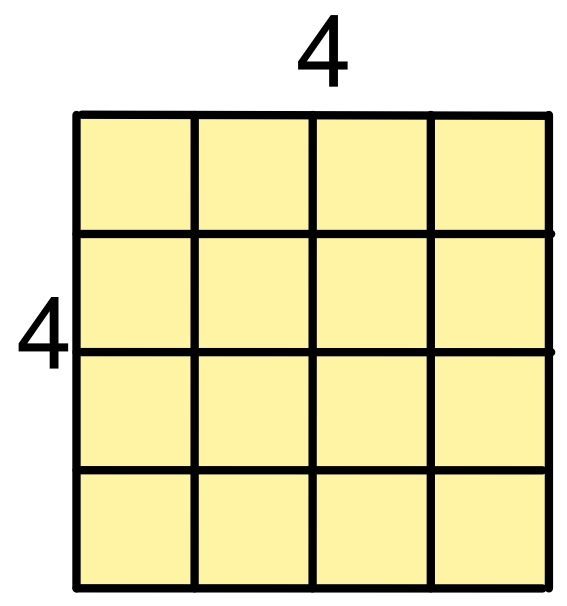Squares

## Squares

Multiplying a number by itself gives the square of a number.

Instead of writing 4 x 4, it is written as 42: the little 2 is an index and means that the 4 should be multiplied by itself. This is read as 4 squared.Any number can be squared. Squaring a negative number will result in a positive number (minus times a minus is a plus):

-1.62 = -1.6 x -1.6 = +2.56.

Squaring a number smaller than 1 will result in an even smaller number:

0.32 = 0.3 x 0.3 = 0.09.

Fractions can also be squared: (frac(1)(2))2 = frac(1)(2) x frac(1)(2) = frac(1)(4).

## Example 1

Calculate 122.

122 = 12 x 12 = 144